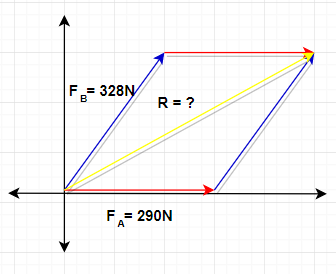# Two dogs pull horizontally on ropes attached to a post; the angle between the ropes is 51.0 deg....

## Question:

Two dogs pull horizontally on ropes attached to a post; the angle between the ropes is 51.0°. Dog A exerts a force of 290 N, and dog B exerts a force of 328 N.

a) Find the magnitude of the resultant force?

b) Find the angle the resultant force makes with the rope of dog A?

Force is known as the push or pulls on an object with mass and this interaction results in a change in velocity of an object. Force is a vector, which means it has both magnitude and direction.

Given:

• Force exerted by Dog A : {eq}F_A = 290\ N{/eq}
• Force exerted by Dog B : {eq}F_B = 328\ N{/eq}
• Angle between the ropes : {eq}\theta = 51^{\circ}{/eq}

Find:

• Magnitude and angle of the resultant force : {eq}F_R =?{/eq} and {eq}\theta= ?{/eq}

To further understand the problem we need to visualize it first using the figure as shown below,(Part a: Magnitude)

We will use the parallelogram Law of vector addition to solve for the resultant force

\begin{align} F_R^2 &= F_A^2 + 2F_A F_B \cos \theta +F_B^2 \\[0.3cm] &= \sqrt {(290\ \rm N)^2 + 2 (290\ \rm N)(328\ \rm N)(cos(51.0^{\circ})) + (328\ \rm N)^2) } \\[0.3cm] &= \sqrt{311405.9 \ \rm N^2} \\[0.3cm] &\approx \boxed{558.0\ \rm N} \end{align}

(Part b: Angle)

To solve for the angle of the resultant force,we will use the formula,

\begin{align} \theta &= \tan^{-1}\left(\frac{\sum y}{\sum x} \right) \\[0.3cm] &= \tan^{-1} \left(\frac{F_B \sin \theta}{F_A +F_B \cos \theta} \right) \\[0.3cm] &= \tan^{-1} \left[ \frac{(328\ \rm N) \sin(51^{\circ})}{(290\ \rm N)+(328\ \rm N) \cos(51^{\circ})} \right] \\[0.3cm] &= \tan^{-1} \left(\frac{258.0}{496.4} \right) \\[0.3cm] &= \tan^{-1}(0.52^{\circ}) \\[0.3cm] &\approx \boxed{27.4^{\circ}} \end{align}